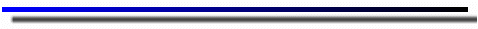# Glossary of terms:

(For use with the Specs Labs)amplitude:

The distance from the midpoint to the crest (or trough) of a wave.

• Image of amplitudes.

angle:

The elevation(y-axis) from ground level(x-axis) that the cannon is pointing.

beats:

A wobbling variation in the loudness of sound caused by interference when two tones of slightly different frequencies are sounded together.

compression:

In sound, a pulse of compressed air or other matter.

• Image of compression in a spring.

constructive interference:

The adding together of two or more waves to form a third wave that has a greater amplitude than either of the orginal waves.

• Image of total constructive interference.
• Image of constructive interference.

destructive interference:

The adding together of two or more waves to form a third wave that has a lesser amplitude than either of the orginal waves. In total destructive interference in sound waves no sound is heard

• Image of total destructive interference.
• Image of destructive interference.

drag:

The friction due to air resistance for an object.

electromagnetic spectrum of waves:

The electromagnetic spectrum is the range or regions into which the the electromagentic waves areclassifyed based on their frequencies or wavelengths.

• Image of electromagnetic specturm.

frequency:

The number of to and fro vibrations of an object, such as a vibrating pendulum or object on a spring.

• Image of changing frequency.

fundamental frequency(wave):

Relating to sound, the lowest frequency. The frequency that determines the pitch of a note.

index of refraction:

This refers to the ratio speeds for light. It is a dimensionless number usually written as

n = speed of light in a vacuum/speed of light in a medium = c/v

interference:

The point where two waves intersect. At this point the waves will add together to form a third wave.

longitudinal wave:

A wave in which the individual particles of a medium vibrate back and forth in the direction in which the wave travels.

• Image of longitudinal wave in a spring.
• Comparision between longitudinal and transverse waves.

phase angle:

The angle between the wave source and the object it interacts. Example a phase angle of 180 degrees means that the two waves are heading towards each other head on.

pitch:

How high or low the wave frequency appears.

Polarization:

When something get polarized this means it has an orientation or preferential direction. If we are talking about light we are refering to the orientation of the transvese oscillations. An object can be unpolarized, partially polarized , or plane (linearly) polarized.

range:

The distance an object has traveled in the x direction.

rarefaction

The phenomenon that occurs when the transmitted wave moves in a direction different than that of the incident wave.

• Image of rarefaction in a spring.

single slit interference:

Wave pattern as wave passes through a single slit opening.

• Image of single slit wave front.

superposition:

The adding together of two waves to form a third wave. This wave will no longer exist as the two original waves continue on their way.

• Image of superposition of two waves.

transverse wave:

A wave in which the individual particles of a medium vibrate from side to side perpendicularly (transversely) to the direction in which the wave travels. The vibrations along a stretched string are transverse waves.

• Image of transverse wave in a spring.
• Image of water drop producing a wave.
• Discription of transverse wave.

velocity:

How fast and in what direction something is travelingEquations:g=acceleration due to gravity.

Distance traveled when motion is uniformed: x=vt

Speed: v=x/t

Vertical distance fallen after any time t: y=1/2(gt2)

Falling speed v after time t: v=gt

Components of Displacement(constant acceleration only)

x=vxot + 1/2axt2

y=vyot + 1/2ayt2

Components of Velocity:

vx=vxo + axt

vy=vyo + ayt

Components of Initial Velocity

vxo = vocostheata

vyo = vosintheata

Projectile Range: (y(initial)=y(final) only)

R = vo2sin(2theata)/g